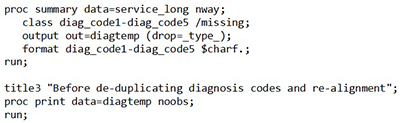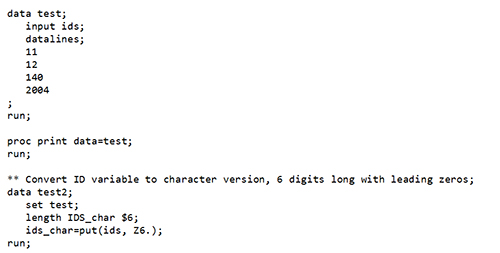## FORMAT Statement:

The format statement associates formats with variables by value.

Syntax:

• FORMAT variable-1 <. . . variable-n> ;
• FORMAT variable-1 <. . . variable-n> format variable-1 <. . . variable-n> format;

Example:

In the example below, the diagnosis code variables are formatted to blank or non-blank values because the actual value is unimportant. Instead, we want to see how the array of five diagnosis codes are arranged. We found using this code that sometimes diag_code1 may be blank, but diag_code2 or diag_code3 may contain values.## PUT Function:

The PUT function is very similar to the PUT statement. Programs use the PUT function to create new variables and using the format to assign values to the new variable.

Syntax:
PUT(source, format.)

Example:

Creating A Character Variable from A Numeric Variable:
The PUT function is commonly used to convert a numeric value into a character value. The following example will create a character variable and adding leading zeroes to a numeric variable using a SAS defined format.** Create test dataset with numeric ID variable;